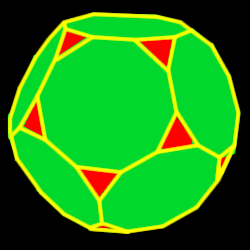# Truncated Dodecahedron

Properties of the truncated dodecahedron: Number of faces, edges and dihedral angle measure

The truncated dodecahedron is created by truncating (cutting off) the tips of the dodecahedron one third of the way into each edge32 total faces:
20 equilateral triangles and 12 decagons

60 vertices:
2 decagons and 1 triangle

90 edges

Dihedral angles
116 degrees, 34 minutes for the dec-dec angle,
142 degrees, 37 minutes for the dec-tri angle